# Difference between AI vs ML vs DL vs DS

Thirumala Reddy

### Last Updated

December 13, 2022

Seclect Blog CategoryAI(Artificial Intelligence):-

AI is an application that is able to do its own task without any human interaction

eg:- Netflix movie recommendation system, and Amazon recommendation system for buying products.

ML(Machine Learning):-

Machine learning is a field of artificial intelligence (AI). Machine learning deals with the concept that a computer program can learn and adapt to new data without human interference by using different algorithms.

DL(Deep Learning):-

Deep learning is nothing but a subset of machine learning that uses algorithms to reflect the human brain. These algorithms that come under deep learning are called artificial neural networks.

DS(Data Science):-

Data science is the study of data. The role of a data scientist involves developing the methods of recording, storing, and analyzing data to effectively extract useful information. The Final goal of data science is to gain insights and knowledge from any type of data.

Let's discuss Machine learning

Machine Learning is divided into 3 types

1)Supervised Machine Learning

2)Un Supervised Machine Learning

3)Reinforcement Machine Learning1)Supervised Machine Learning:-

Supervised Machine Learning has 2 types

1)Classification

2)Regression

Classification:-

-->Classification is a process of categorizing a given data into different classes.

-->Classification can be performed on both structured and unstructured data to categorize data.

eg:-Classifying the mail whether it belongs spam or not spamRegression:-

Regression models are used for prediction on a continuous value.
eg:-Predicting prices of a house given the features  like size, price, area of location etc.
UN-SUPERVISED MACHINE LEARNING:-
Unsupervised machine learning is nothing but it uses machine learning algorithms to analyze the data and cluster the unlabeled datasets. There are no dependent variables in Un Supervised Machine Learning
Un Supervised machine learning is divided into 2 types
1)Clustering
2)Dimensionality Reduction
1)Clustering:-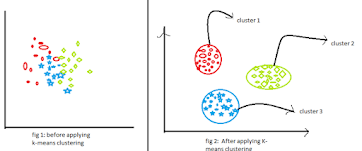eg:-I have a company & I want to release 2 products,1st product is costly so I want to target Rich people, 2nd product is the medium cost so I want to target middle-class people. So when I am doing Add Marketing I can apply customer segmentation & can focus on that particular clusters

2)Dimensionality Reduction:-
When we have imbalanced data i.e. for example 800 women & 200 men.When the new data point enters there is a high chance that the new data point may be grouped under the women cluster. This is main problem with imbalanced data. So by using dimensionality reduction concepts we will decrease or increase the data points.
The algorithms that will come under supervised machine learning are:-
1)Linear Regression
2)Ridge & Lasso Regression
3)Logistic Regression
4)Decision Tree
6)Random Forest
8)Xg Boost
9)Naïve Bayes'
10)SVM
The algorithms that will come under Un-Supervised machine learning are:-
1)K means
2)DB Scan
3)Hierarchical clustering
4)K nearest neighbor cluster
5)PCA

LINEAR REGRESSION
In Linear Regression we are trying to find out the best fit line which will help us to do a prediction
y =  MX + c+ error term
where
y=Dependent variable
x=Independent variable
m=co-efficient or slope
c=Intercept

Residuals are nothing but the difference between the Actual Response & Predicted Response points.
-->The line which has a sum of Residuals very less is called BEST FIT LINE.
-->We need to select a linear regression model which has fewer residuals & we need to minimize the sum of square residuals.
y=mx + c+ error term
Here mx +c is the explained variance
The error term is un-explained variance
Performance Metrics:-
Performance metrics are used to verify how good our model is with respect to linear regression.
There are 2 types of performance metrics
1)R^2(Co-efficient of Determination)
1)Co-efficient of Determination:--
The coefficient of Determination is the matrix that explains to us the extent the explained variance affects y. Co-efficient of Determination is given by R^2
--->R^2 lies between 0 to 1.
--->Higher value of R^2 represents that the Predicted Response is near to the Actual Response.= coefficient of determination= the sum of squares of residuals= the total sum of squares
-->The top left chart of the above fig shows a linear regression line that has a very low ?² indicating predicted responses by our mode are no way near to actual responses.
This is an example of an underfitting condition.

-->The top right chart of the above fig indicates the polynomial regression with a degree equal to 2.

-->The bottom left chart of the above fig indicates a polynomial regression with a degree equal to 3.
The value of ?² is higher when compared to preceding cases.
This model behaves better with known data when compared with the previous ones.
--> In the bottom right plot, we can observe the value of R^2=1, indicating that predicted responses are equal to actual responses.
In many cases,  this is an overfitted model.

-->R^2 will consider all values, R^2 won't care about whether values will affect output or not. So it will consider unnecessary values & predict R^2 but Adjusted R^2 will consider only required data for example

• R^2 will consider DOB, No of years of Experience & Degree to predict the salary of a person but whereas
•  Adjusted R^2 will consider The years of Experience & Degrees to predict the salary of a person
-->Every time we add an independent variable to a model the R^2 increase's even if the independent variable is insignificant. Whereas adjusted R^2 increase's only when the independent variable is significant & affects the dependent variable
-->Adjusted R^2 value always be less than or equal to the R^2 value.
COST FUNCTION:-
The cost function is the technique of evaluating "the performance of our algorithm/model". It takes both the predicted output by the model and the actual output and calculates how much wrong the model was in its prediction. It outputs a higher number if our predictions differ a lot from the actual values.
Gradient Descent acts like an optimization algorithm that is used for finding a local minimum. Gradient descent is used to find the parameters that help us to minimize the cost function as for as possible.
Overfitting is nothing but the with respect to training dataset getting low error & with respect to testing data getting high error & high variance. i.e. Our model performed well with training data & fails to perform well with test data.
Underfitting:-
Underfitting is nothing but getting a high error with respect to both the training dataset & testing dataset.  i.e. our model  Accuracy will be bad with respect to both training data & testing data
-->If we get low Bias & low variance then the model is called a Generalized model.
-->In simple words
Low bias & High variance-->Overfitting
High bias & High variance-->Underfitting
Low bias & Low variance-->Generalized Model
-->By using RIDGE & LASSO we convert high variance to low variance.
RIDGE & LASSO REGRESSION

### Ridge Regression

• Ridge regression is similar to linear regression, but in ridge regression, a small amount of bias is introduced to get better long-term predictions.
• Ridge Regression will prevent overfitting, so the output of Ridge Regression we get is a generalized model.
• Ridge regression is also called L2 regularization.
• The penalty which we added to the cost function is called the Ridge Regression penalty. The penalty can be calculated by multiplying the lambda by the squared weight of each individual feature.
• In Ridge regression, the co-efficient value(λ) will come near to 'zero' but won't become 'zero'
• Ridge Regression is preferred for small & medium dimensionality data.
• The equation for the cost function in ridge regression is as follows:• Lambda(λ) value is selected by using cross-validation.

### Lasso Regression:-

• Lasso Regression is also known as L1 Regularization.
• Lasso Regression helps in

1)Preventing Overfitting
2)Perform Feature Selection

• Lasso regression is a type of linear regression but Lasso Regression uses shrinkage.
• Lasso Regression is preferred when we have high dimensions in data
• In Lasso Regression the co-efficient value may become 'zero'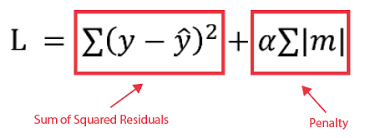• In the above formula, we can observe that there was no square to penalty so the features which are not important are not squared up as of Ridge Regression. So, the value of features that are not important won't increase. In short in Lasso Regression we are reducing the value of the cost function by performing the  feature selection by not increasing the value of features that are not important

Assumption of Linear Regression:-

1. We Assume that the data follows Normal/Gaussian Distribution
2. Scaling(Standardization) of the data is done
3. Assuming the data follows Linearity
4. Assuming multi-collinearity does not exist. If exist drop one of the highly co-related feature.

LOGISTIC REGRESSION
• The logistic Regression algorithm is used for classification problems
• There are two types of problems statement's in Logistic Regression

1)Binary Classification

2) Multi-Class Classification
Logistic regression is a popular Machine Learning algorithms, that comes under the Supervised Learning category. It is used for predicting the categorical dependent variable using a given set of independent variables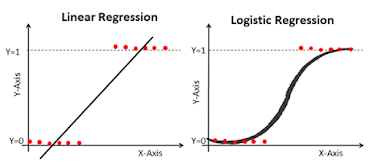from the above figure we can observe that in Linear Regression if outlier is present the best fit line changes which result in mis-classification of data if we use Linear Regression for classification. It is not in the case of Logistic Regression. In Logistic Regression we curve will be in the shape of  "S", not as like as a "Line" as per the Linear Regression . So, as the curve shape is "S", the classification of data points will occur accurately.  So, for classification of data ,Logistic Regression is used.

CROSS-VALIDATION:-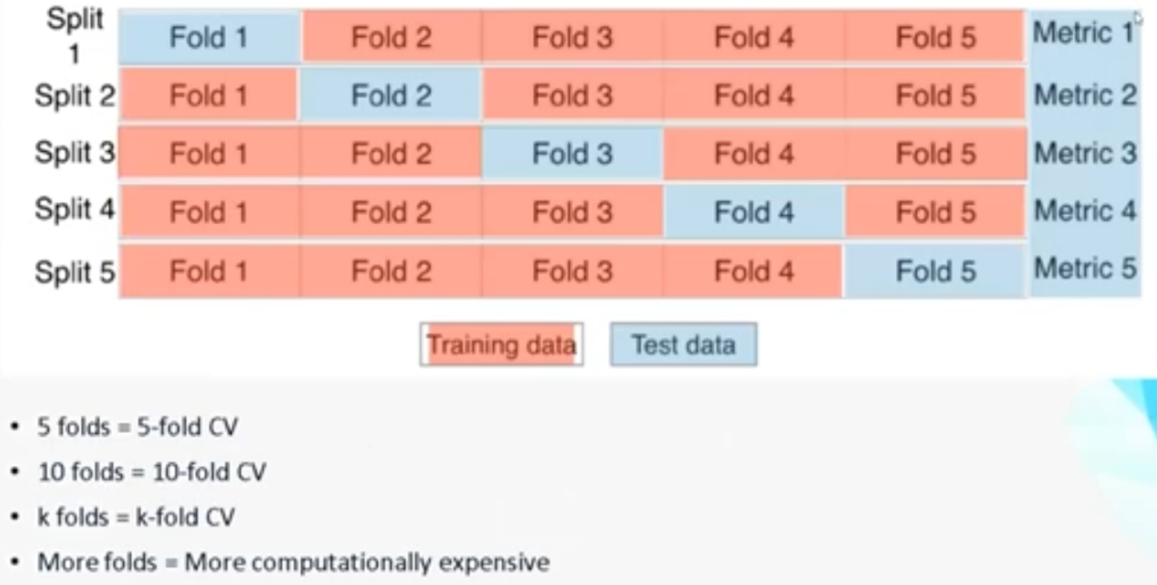• we use cross-validation for  not depending on only 1 split, creating multiple splits of data i.e. 5 parts.1part is used for training, remaining for testing, 2nd time 2part is used for training & remaining for testing...…..
• For every round we will calculate the error matrix i.e. root mean square error & then mean of all values it is cross-validation
• No of folds as we can wish, but we mostly use 5,10 . 10 is most prefered. Size of data is also matters for small data 10folds are not preferred.
• Similarly we have have different ways .They are
Leave one out cross validation:--
in this for example we have 1000values in that 1st time 1st values for testing remaining for training, 2nd time 2nd value for testing remaining for training this will continue for every element so this way every single observation is acting as a test data at one point of time & remaining 999 will go to training. More computation time. Now a days no one is using
Repeated k fold:-- Repeating the process .i.e.  we divide data in 5folds and repeat the same 1st process 3times. I.e. 1st done, 2nd time data is shuffled. It good in some cases that when we feel 1st process is alone not good
Nested k fold or double k fold:--If we are running 5 folds, now each fold will again do 5 loops. I.e. Inside every loop their will be again 5loops
Stratified K fold Cross Validation:--The major disadvantage of k fold is for example we have 600 Yes,400 No, in the first fold their is a chance of only yes may present. So we wont get proper accuracy of a model. But Stratified K fold Cross Validation make sure that the all class values are present
Time Series Cross Validation:- For example consider stock value prediction. Based on the day1 to day5 values stock value predicted of day6. & based on the day2 to day6 values,7th day is predicted & day3 to day7,day 8 is predicted this continues this is called Time Series Cross Validation
PERFORMANCE METRICS:-
Performance metrics are used to find out how well our Model is working
1)Confusion Matrix:-

A confusion matrix is one of the way to evaluate the performance of our algorithm. To construct confusion matrix we will take both predicted & actual responses of our model & we construct confusion matrix as below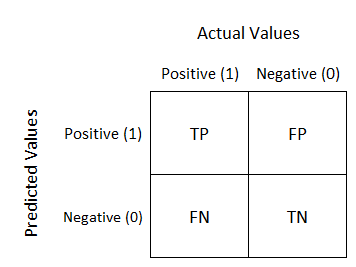where

TN(True Negatives) - model predicts negative outcomes and the real/known outcome is also negative
TP(True Positives) - Model predicts positive outcome and the real outcome is also positive
FN(False Negatives) - model predicts negative outcome but known outcome is positive
FP(False Positives) - model predicts positive outcome but known outcome is negative
2)ACCURACY:--
Accuracy is one metric for evaluating classification models. It is the ratio of  Number of correct predictions to the Total number of predictions

-->Generally the result of accuracy is taken into consideration for Balanced data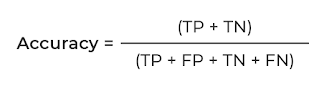3)PRECISION:--
Precision can be defined as out of total actual predicted positive values, how many values are actually positive is called Precision.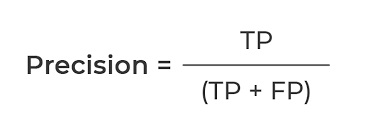• Whenever FP is more important to reduce use Precision

eg:-In Spam classification, if we got spam mail it should be identified as spam & in spam classification we should concentrate on reducing FP i.e. even though the mail we got is not a spam but if our algorithm detects it as a spam, then we are going to miss our important mails .so in order to avoid this case we should concentrate on reducing FP

4)RECALL:--

Recall can be defined as out of total actual positive values, how many values did we correctly predicted positive is called Recall.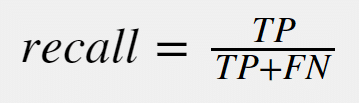• When ever FN is more important to reduce use Recall

eg:-In classifying a person whether we has cancer or not FN is more important to reduce. If our model predicts that a person don't have a cancer even though  he has a cancer this leads to increase of cancer cells in his body & affects his health.

5)F-BETA:--
The F-beta score is nothing  the harmonic mean of both precision and recall. If the result of F-Bets is near or equal to 1 means the model is performing good. If the result of F-Beta is near or equal to zero means we can conclude that the model is not at all performing good.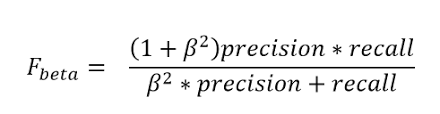->When ever if we want to reduce both FP & FN  use β=1.It is also called as F1 Score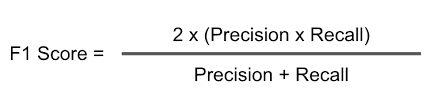-->When ever FP is more important to reduce use β=0.5
• F0.5-Measure = ((1 + 0.5^2) * Precision * Recall) / (0.5^2 * Precision + Recall)
• F0.5-Measure = (1.25 * Precision * Recall) / (0.25 * Precision + Recall)
-->when ever FN is more important to reduce use β=2
• F2-Measure = ((1 + 2^2) * Precision * Recall) / (2^2 * Precision + Recall)
• F2-Measure = (5 * Precision * Recall) / (4 * Precision + Recall)
NAIVE BAYES
Bayes Theorem:-
Naive Bayes algorithm is used for Classification, Which works on Bayes Theorem.The formula of the Bayes theorem is given below.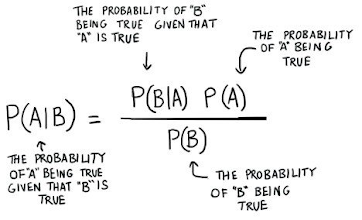#### Working of Naïve Bayes' Classifier:

Lets understood how the naive Bayes classifier will work by using below example:

Let's take the dataset of weather conditions and the corresponding target variable "Play". In the different, we have records of different whether conditions and with respect to the corresponding weather condition whether he/she can play or not.

Now by using the dataset we are classifying whether he/she can play when weather is sunny

Solution: To solve this, first consider the below dataset:

 Outlook Play 0 Rainy Yes 1 Sunny Yes 2 Overcast Yes 3 Overcast Yes 4 Sunny No 5 Rainy Yes 6 Sunny Yes 7 Overcast Yes 8 Rainy No 9 Sunny No 10 Sunny Yes 11 Rainy No 12 Overcast Yes 13 Overcast Yes
Frequency table for the Weather Conditions:
 Weather Yes No Overcast 5 0 Rainy 2 2 Sunny 3 2 Total 10 5

Likelihood table weather condition:

 Weather No Yes Overcast 0 5 5/14= 0.35 Rainy 2 2 4/14=0.29 Sunny 2 3 5/14=0.35 All 4/14=0.29 10/14=0.71

Applying Bayes'theorem:

P(Yes|Sunny)= P(Sunny|Yes)*P(Yes)/P(Sunny)

P(Sunny|Yes)= 3/10= 0.3

P(Sunny)= 0.35

P(Yes)=0.71

So P(Yes|Sunny) = 0.3*0.71/0.35= 0.60

P(No|Sunny)= P(Sunny|No)*P(No)/P(Sunny)

P(Sunny|NO)= 2/4=0.5

P(No)= 0.29

P(Sunny)= 0.35

So P(No|Sunny)= 0.5*0.29/0.35 = 0.41

From above result we can notice that P(Yes|Sunny)>P(No|Sunny)

Hence on a Sunny day, Players can play the game.

K-Nearest Neighbor

K-Nearest Neighbor (KNN) is a supervised machine learning algorithm used for solving both classification and regression problems.
-->KNN algorithm is easy to implement
-->The major drawback of the KNN algorithm is it becomes slow when the size of the data is large.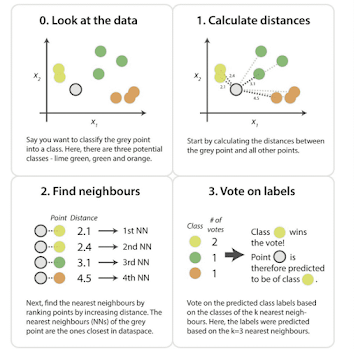-->KNN works on the distance concept

-->As shown in the above figure the distance is calculated from the point that we want to classify into categories to all nearby points. After finding out the neighbors, voting is done  & based on the no of votes got, the point is classified into any category. This is for the Classification
--->For Regression type of problems, if we give K=5, where K is the hyperparameter, 5 nearest points average is calculated. The average of all points is the output.
--> There are two ways to calculate the distance between two points
1)Euclidean Distance
2)Manhattan Distance
Euclidean Distance:-
Let us consider two points A(X1,Y1)  & B(X2,Y2).The Euclidean distance formula to measure the distance between these two A & B is as follows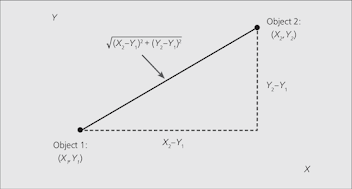Manhattan Distance:-

Let us consider two points A(X1,Y1)  & B(X2,Y2).The Manhattan Distance formula to measure the distance between these two A & B is as follows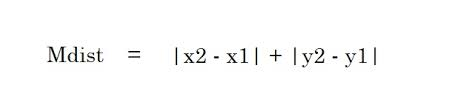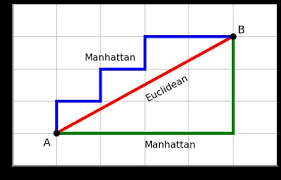Assumptions of K-Nearest Neighbor:-

1)KNN assumes that the outliers are not present in the data
2)KNN assumes that the data is balanced data
LET'S DISCUSS SOME OF THE OTHER CONCEPTS BEFORE WE MOVE INTO THE NEXT ALGORITHM
Handling Imbalanced Data:-
Imbalanced data refers to those types of datasets where the target class has an uneven distribution of observations, i.e. one class label has a very high number of observations and the other has a very low number of observations.
--> There are two ways to handle imbalance data
1)By Under Sampling method
2)By Over Sampling method
1)Under Sampling:-
Undersampling is a technique to balance uneven datasets by keeping all of the data in the minority class and decreasing the size of the majority class.
eg:- If we have 900-->Yes & 100-->No in the dataset, It is an imbalance dataset in order to balance the data by using under-sampling 900 Yes is decreased to 100 Yes
-->Under Sampling is done only when we have a huge dataset
-->In most cases Under Sampling is not preferred as we are going to lose the data
2)Over Sampling:-
Random oversampling involves randomly selecting examples from the minority class, with replacement, and adding them to the training dataset.
-->In oversampling the chances of overfitting may occur.
-->In Over Sampling we won't decrease the records instead we increase the no of records
eg:-If we have 900-->Yes & 100-->No in the dataset, by using Over Sampling we try to increase the 100-->No to 900 No
-->Over Sampling is the most preferred method if we have a small dataset
CURSE OF DIMENSIONALITY:-
As the no of features increases, the accuracy of the model increases but as the no of features increases exponentially(greatly), the model gets confused because we are feeding a lot of information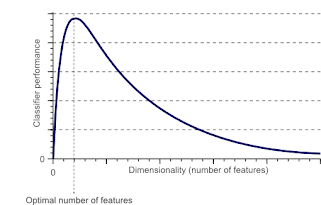-->From above we can observe as the no of features increased the performance of the model decreased.

Principal component analysis:-
Principle Component Analysis(PCA) is an unsupervised machine learning algorithm.PCA is used to decrease to no of dimensions.
--> Let's consider an example of why we need to decrease the no of dimensions
We have a dataset of salary prediction. The columns in the dataset are No of Years of Experience, Current CTC, Highest Qualification, and D.O.B in this dataset D.O.B is not required to predict salary. So we can remove the D.O.B Column. If the no of dimensions is fewer means machine learning can perform well.
DECISION TREE
A decision tree is the most powerful and popular tool for classification and prediction. A Decision tree is a flowchart-like tree structure, where each internal node denotes a test on an attribute, each branch represents an outcome of the test, and each leaf node (terminal node) holds a class label.
• A Decision Tree is used to solve both classification & Regression types of Problems.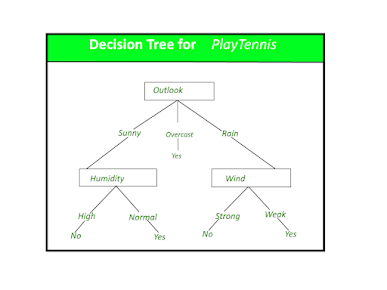Leaf Node:
The leaf nodes (green), also called terminal nodes, are nodes that don't split into more nodes.

-->A node is 100% impure when a node is split evenly 50/50 and 100% pure when all of its data belongs to a single class. In order to optimize our model we need to reach maximum purity and avoid impurity.

In the decision Tree, the purity of the split is measured by

1)Entropy

2)Gini Impurity

The features are selected based on the value of Information Gain

1)Entropy

-->Entropy helps us to build an appropriate decision tree for selecting the best splitter.

-->Entropy can be defined as a measure of the purity of the sub-split.

-->Entropy always lies between 0 to 1.

-->The entropy of any split can be calculated by this formula.

-->The split in which we got less entropy is selected & proceeds further

Information Gain:-

Information gain is the basic criterion to decide whether a feature should be used to split a node or not. The feature with the optimal split i.e., the highest value of information gain at a node of a decision tree is used as the feature for splitting the node

--->Information Gain is calculated for a split by subtracting the weighted entropies of each branch from the original entropy. When training a Decision Tree using these metrics, the best split is chosen by maximizing Information Gain.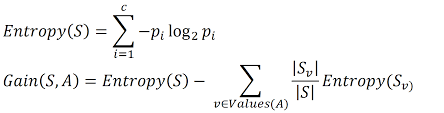-->The feature for which we got higher Information Gain is selected & proceed further.

Gini impurity:--
Gini impurity is a function that determines how well a decision tree was split. Basically, it helps us to determine which splitter is best so that we can build a pure decision tree.
-->Gini impurity ranges values from 0 to 0.5.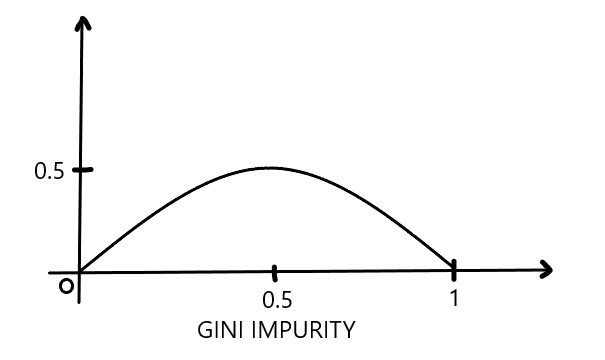-->Gini impurity has a maximum value of 0.5, which is the worst we can get, and a minimum value of 0 means the best we can get.

-->Gini impurity is faster than entropy. If we have large data Gini impurity is most preferred.
##### -->The benefit of a continuous variable decision tree is that the outcome can be predicted based on multiple variables rather than on a single variable as in a categorical variable decision tree
-->Based on the Information Gain values the feature for splitting is selected
--->If we have millions of records time of computation is very very high. i.e. as no of records increases the of computations increases. SO a decision tree for continuous values, if we have large data, is not preferred.

### Decision Tree - Regression

A decision tree builds regression or classification models in the form of a tree structure. It breaks down a dataset into smaller and smaller subsets while at the same time an associated decision tree is incrementally developed. The final result is a tree with decision nodes and leaf nodes. A decision node (e.g., Outlook) has two or more branches (e.g., Sunny, Overcast, and Rainy), each representing values for the attribute tested. The leaf node (e.g., Hours Played) represents a decision on the numerical target. The topmost decision node in a tree corresponds to the best predictor called the root node. Decision trees can handle both categorical and numerical data.

-->Based on the mean squared error(MSE) the splitting done in regression type of problems in the Decision TreeThe strengths of decision tree methods are:

• Decision trees are able to generate understandable rules.
• Decision trees perform classification without requiring much computation.
• Decision trees are able to handle both continuous and categorical variables.
• Decision trees provide a clear indication of which fields are most important for prediction or classification.

The weaknesses of decision tree methods :

• Decision trees are less appropriate for estimation tasks where the goal is to predict the value of a continuous attribute.
• Decision trees are prone to errors in classification problems with many classes and a relatively small number of training examples.
• A decision tree can be computationally expensive to train. The process of growing a decision tree is computationally expensive. At each node, each candidate splitting field must be sorted before its best split can be found. In some algorithms, combinations of fields are used and a search must be made for optimal combining weights. Pruning algorithms can also be expensive since many candidate sub-trees must be formed and compared.

#### Hyperparameter tuning:-

As the no of splits increases in the decision tree. The model leads to overfitting. In order to avoid overfitting in the decision Tree there are 2 methods
1)Post-Pruning
2)Pre-Pruning
In general, pruning is a process of removal of selected parts of plants such as buds, branches, and roots. Decision Tree pruning does the same task it removes the branches of the decision tree to overcome the overfitting condition of the decision tree.
1)POST-PRUNING:-
• This technique is used after the construction of the decision tree.
• This technique is used when the decision tree will have a very large depth and will show overfitting of the model.
• It is also known as backward pruning.
• This technique is used when we have infinitely grown decision trees.
• Here we will control the branches of a decision tree that is max_depth and min_samples_split using cost_complexity_pruning

2. Pre-Pruning:-
• This technique is used before the construction of a decision tree.
• Pre-Pruning can be done using Hyperparameter tuning.
• Overcome the overfitting issue.

In this blog, i will use GridSearchCV for Hyperparameter tuning.

Ensemble Techniques
Ensemble methods are techniques that create multiple models and then combine them to produce improved results. Ensemble methods usually produce more accurate solutions than a single model would. This has been the case in a number of machine learning competitions, where the winning solutions used ensemble methods.
-->Ensemble Techniques are of 2 types
1)Bagging(Bootstrap Aggregation)
2)Boosting
Bagging:-
Bagging, also known as bootstrap aggregation, is the ensemble learning method that is commonly used to reduce variance within a noisy dataset. In bagging, a random sample of data in a training set is selected with replacement—meaning that the individual data points can be chosen more than once. After several data samples are generated, these weak models are then trained independently, and depending on the type of task—regression or classification, for example—the average or majority of those predictions yield a more accurate estimate.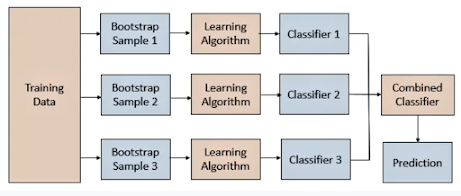In simple words Bagging means the training data is divided into multiple small datasets & we train each small datasets different algorithms were applied & result is drawn. For classification the voting is done on the results i.e. we will go with the results which got the highest votes. For Regression, the mean of the results is taken
-->The algorithms that come under bagging are
1)Random Forest Classifier
2)Random forest Regressor
Random Forest Classifier & Random forest Regressor:-
In the Decision Tree, the problem of overfitting occurs i.e. Low Bias & High Variance. By using Random Forest we convert High Variance to Lower Variance.

Random Forest is a popular machine learning algorithm that belongs to the supervised learning technique. It can be used for both Classification and Regression problems in ML. It is based on the concept of ensemble learning, which is a process of combining multiple classifiers to solve a complex problem and improve the performance of the model.

As the name suggests, "Random Forest is a classifier that contains a number of decision trees on various subsets of the given dataset and takes the average to improve the predictive accuracy of that dataset." Instead of relying on one decision tree, the random forest takes the prediction from each tree and based on the majority votes of predictions, predicts the final output.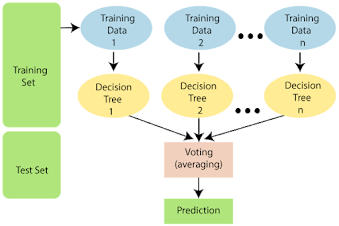Boosting:-
In machine learning, boosting is an ensemble meta-algorithm for primarily reducing bias, and also variance in supervised learning, and a family of machine learning algorithms that convert weak learners to strong ones.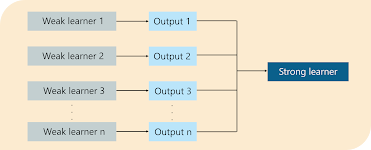-->The algorithms that come under Boosting are

3)Xgboost
AdaBoost (Adaptive Boosting) is a very popular boosting technique that aims at combining multiple weak classifiers to build one strong classifier.
-->A single classifier may not be able to accurately predict the class of an object, but when we group multiple weak classifiers with each one progressively learning from the others' wrongly classified objects, we can build one such strong model.
-->The Decision trees with only 1 split are called Decision Stumps.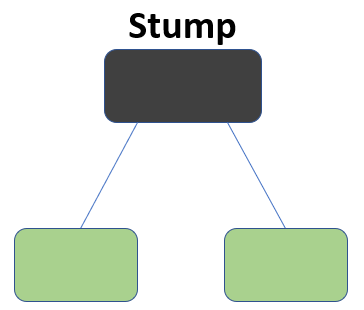#### Understanding the working of the AdaBoost Algorithm:-

Step 1:-calculate the sample weights

The formula to calculate the sample weights is:

Where N is the total number of data points

Step2:-creating a decision stump

We’ll create a decision stump for each of the features and then calculate the Gini Index of each tree. The tree with the lowest Gini Index will be our first stump.

Step3:-Calculating Performance say

We’ll now calculate the “Amount of Say” or “Importance” or “Influence” for this classifier in classifying the data points using this formula: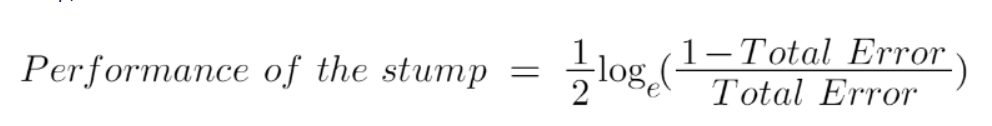Step4:- Updating the weights

we need to update the weights because if the same weights are applied to the next model, then the output received will be the same as what was received in the first model.

The wrong predictions will be given more weight whereas the correct predictions' weights will be decreased. Now when we build our next model after updating the weights, more preference will be given to the points with higher weights.

After finding the importance of the classifier and total error we need to finally update the weights and for this, we use the following formula:

Step5:-Creating the buckets

We will create buckets based on Normalized weights

#### -->XGBoost is used for both classification & Regression

-->XGBoost is a decision tree-based ensemble Machine Learning algorithm that uses a gradient boosting framework.
-->In this algorithm, decision trees are created in sequential form. Weights play an important role in XGBoost. Weights are assigned to all the independent variables which are then fed into the decision tree which predicts results. The weight of variables predicted wrong by the tree is increased and these variables are then fed to the second decision tree. These individual classifiers/predictors then ensemble to give a strong and more precise model. It can work on regression, classification, ranking, and user-defined prediction problems.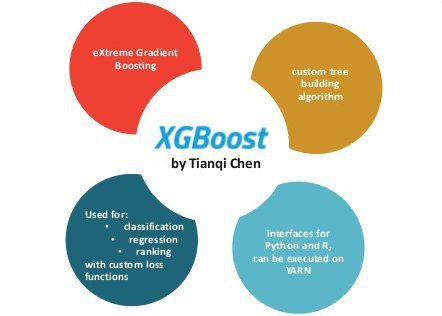Support Vector Machine(SVM):-
Support Vector Machine(SVM) is a supervised machine learning algorithm used for both classification and regression problem statements.
--> Margin line is drawn at the point where the distance is minimal from the origin line i.e. line is drawn at the point is near to origin line
-->Always should consider the line as a more marginal distance so that all data points can be divided properly.
-->From the above fig, it is clear that we will choose a large margin distance one.

-->The classification of data points can be done by using a single line or it can also be done by using the nonlinear line. For linear we use kernel="linear" and for nonlinear line we use kernel="rbf"

Un-Supervised Machine Learning
The algorithm's that we will discuss in unsupervised Machine Learning are
1)K Means Clustering
2)Hierarichal Clustering
3)Silhouette Score
4)DBScan Clustering
K Means Clustering:-
K-Means is a technique for data clustering that may be used for unsupervised machine learning. It is capable of classifying unlabeled data into a predetermined number of clusters based on similarities (k).
-->The K-means clustering algorithm computes centroids and repeats until the optimal centroid is found.
-->In this method, data points are assigned to clusters in such a way that the sum of the squared distances between the data points and the centroid is as small as possible.

Below are some of the features of K-Means clustering algorithms:

• It is simple to grasp and put into practice.
• K-means would be faster than Hierarchical clustering if we had a high number of variables.
• An instance’s cluster can be changed when centroids are re-computation.
• When compared to Hierarchical clustering, K-means produces tighter clusters.

Some of the drawbacks of K-Means clustering techniques are as follows:

• The number of clusters, i.e., the value of k, is difficult to estimate.
• A major effect on output is exerted by initial inputs such as the number of clusters in a network (value of k).
• The sequence in which the data is entered has a considerable impact on the final output.
• It’s quite sensitive to rescaling. If we rescale our data using normalization or standards, the outcome will be drastically different. ultimate result
• It is not advisable to do clustering tasks if the clusters have a sophisticated geometric shape.
Hierarchical Clustering:-
Hierarchical clustering is an unsupervised machine-learning algorithm used for forming clusters. If we have large dataset K Means is preferred but if we have a small dataset Hierarchal Clustering is preferred. In Hierarchical clustering, we construct a dendrogram. Let's see how to construct a dendrogram

-->Let’s take six data points A, B, C, D, E, and F for constructing a dendrogram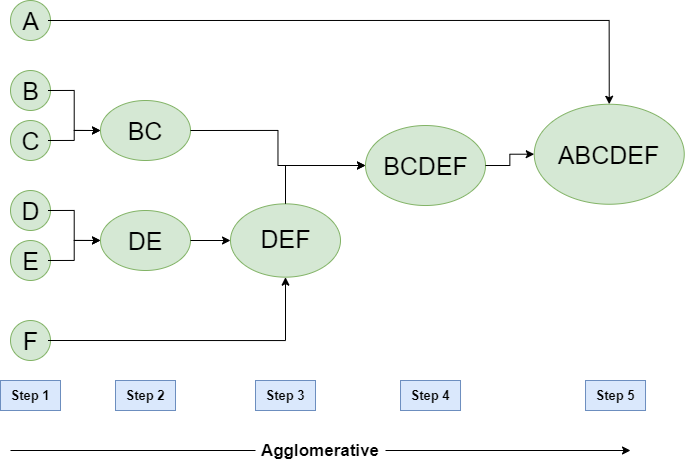• Step-1:
In Step -1 let's assume each data point is a separate cluster and we calculate the distance between one cluster to each and every cluster.
• Step-2:
In Step 2 based on the distance between cluster, we start grouping clusters. From the above example, we can observe that B and C are nearer to each other. so we form BC as one cluster and D, and E are near to each other so we form DE as one cluster
• Step-3:
In Step 3 again we calculate the distance between the cluster BC, DE, and F and we observed the cluster DE and F are near to each other. So we will form DEF as one cluster.
• Step-4:
In Step-4 again we repeat the same above process and BC, and DEF are grouped as one cluster as BCDEF
• Step-5:
In Step 5, the two remaining clusters are merged together to form a single cluster as ABCDE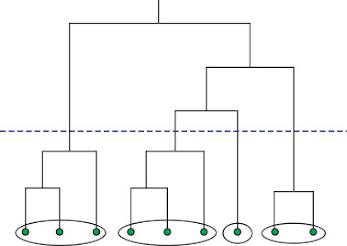-->We need to find the longest vertical line that has no horizontal line passed through it & K value is nothing but the no of intersections that the horizontal line has
-->In the above fig, we can observe that the longest vertical line that has no horizontal line passes through it has 4 intersections. So, the K value is 4

Silhouette Clustering:-

-->Silhouette Clustering is used to validate the K Means & Hierarchical Clustering Model.
-->Silhouette refers to a method of interpretation and validation of consistency within clusters of data.
-->The silhouette value is a measure of how similar an object is to its own cluster (cohesion) compared to other clusters (separation).
-->The silhouette ranges from −1 to +1, where a high value indicates that the object is well-matched to its own cluster and poorly matched to neighboring clusters.
Assume the data have been clustered via any technique, such as k-means, into clusters.
-->First we need to calculate an (I)
-->a(I) is nothing but the mean distance betweenand all other data points in the same cluster
-->For data point(data pointin the cluster)-->We calculate the distance between i point to all other points in the same cluster i.e. within cluster 1 only & we do the average.
STEP2:-
-->It is the mean distance ofto all points in any other cluster
-->The cluster with this smallest mean dissimilarity is said to be the "neighboring cluster" and is selected as another cluster

-->For each data point, we now define-->We calculate the distance from each point in cluster 1 to each point in cluster 2 & we do an average.
STEP3:-
-->If ai<bi, then it is a Good Cluster
-->If ai>bi, then it is a bad cluster
STEP4:-
-->We now define a silhouette (value) of one data point, if-->Silhouette value ranges from -1 to +1, Where a high value indicated that the object is well matched to its own cluster & poorly matched to neighbor cluster similarly and vice versa.

## DBSCAN Clustering:-

The full form of  DBSCAN is  Density-based spatial clustering. DBSCAN is a unsupervised machine learning algorithm used for performing clustering. DBSCAN was proposed by Martin Ester, Hans-Peter Kriegel, Jörg Sander, and Xiaowei Xu in 1996.
-->Take a point & with Eps(Epsilon) distance as a radius, and draw a circle, if we give MinPts=4 and no of points lying inside the circle are minimum of 4 then it is called as CORE POINT.
-->If at least 1 point is present inside a circle then it is called a Border Point.
-->If no point is present inside the circle then it is called Noise Point.